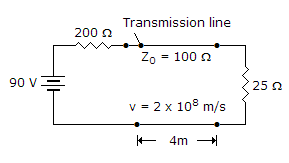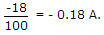# Electronics and Communication Engineering - Microwave Communication

### Exercise :: Microwave Communication - Section 3

6.

The semiconductor diode which can be used in switching circuit in microwave range is

 A. PIN diode B. Varactor diode C. Tunnel diode D. Gunn diode

Explanation:

A PIN diode has an intrinsic (i) layer between p and n layers. When reverse bias is applied depletion layers are formed at p-i and i-n junctions.

The effective/width of depletion layer increases by the width of i layer. It can be used as a voltage controlled attenuator.

At high frequencies the rectification effect ceases and impedance of diode is effectively that of i layer.

This impedance varies with the applied bias. It is used in high frequency switching circuits, limiters, modulators etc.

7.

In the given figure the reflected current wave after first reflection isA. 0.18 A B. -0.18 A C. 0.3 A D. -0.3 A

Explanation:8.

A line has an attenuation of 0.054 Np/m. The attenuation in decibels is

 A. 4.7 dB/m B. 0.47 dB/m C. 0.54 dB/m D. 5.4 dB/m

Explanation:

0.054 x 8.68 = 0.47 dB/m.

9.

If a line is open circuited Zin = Z0 tanh (gl).

 A. True B. False

Explanation:

The quantities required to be measured in microwave circuits are frequency, power and impedance.

Microwave frequency measurement is done by slotted line, resonant cavities and transfer oscillator.

Microwave power measurement uses bolometers and micromave power meters.

A self balancing bridge working on the principle of power substitution is commomly used.

Microwave impedance measurement is done by measurement of reflection coefficient and VSWR.

A slotted line and probe is a basic tool for these measurements.' Network analyser enables rapid impedance measurement over a broad frequency range.

10.

A reflex klystron oscillator uses

 A. one cavity resonator B. two cavity resonators C. three cavity resonators D. none of the above

Explanation:

It uses a single cavity resonator for generating microwave oscillations.

Its parts are electron gun, resonator, repeller and output coupling.

It operates on the principle of positive feed back.

The repeller electrode is at negative potential and sends the partially bunched electron beam back to resonator cavity.

This positive feedback supports oscillations. Its feature are:

1. Frequency range - 2 to 100 GHz

2. Power output - 10 MW to about 2 W

3. Efficiency - 10 - 20 %

Its applications include radar receivers, local oscillator in microwave devices, oscillator for microwave measurements in laboratories etc.